## TCP Congestion Control-

Before you go through this article, make sure that you have gone through the previous article on TCP Congestion Control.

TCP congestion control policy consists of following three phases-1. Slow Start
2. Congestion Avoidance
3. Congestion Detection

In this article, we will discuss practice problems on TCP Congestion Control.

## Problem-01:

The growth of congestion window takes place-

1. Infinitely
2. Up to Threshold
3. Up to the size of receiver’s window
4. Up to timeout

## Solution-

Option (C) is correct.

## Problem-02:

Consider the effect of using slow start on a line with a 10 msec RTT and no congestion. The receiver window is 24 KB and the maximum segment size is 2 KB. How long does it take before the first full window can be sent?

## Solution-

Given-

• Receiver window size = 24 KB
• Maximum Segment Size = 2 KB
• RTT = 10 msec

### Receiver Window Size-

Receiver window size in terms of MSS

= Receiver window size / Size of 1 MSS

= 24 KB / 2 KB

= 12 MSS

### Slow Start Threshold-

Slow start Threshold

= Receiver window size / 2

= 12 MSS / 2

= 6 MSS

### Slow Start Phase-

• Window size at the start of 1st transmission = 1 MSS
• Window size at the start of 2nd transmission = 2 MSS
• Window size at the start of 3rd transmission = 4 MSS
• Window size at the start of 4th transmission = 6 MSS

Since the threshold is reached, so it marks the end of slow start phase.

Now, congestion avoidance phase begins.

### Congestion Avoidance Phase-

• Window size at the start of 5th transmission = 7 MSS
• Window size at the start of 6th transmission = 8 MSS
• Window size at the start of 7th transmission = 9 MSS
• Window size at the start of 8th transmission = 10 MSS
• Window size at the start of 9th transmission = 11 MSS
• Window size at the start of 10th transmission = 12 MSS

From here,

• Window size at the end of 9th transmission or at the start of 10th transmission is 12 MSS.
• Thus, 9 RTT’s will be taken before the first full window can be sent.

So,

Time taken before the first full window is sent

= 9 RTT’s

= 9 x 10 msec

= 90 msec

## Problem-03:

Consider an instance of TCP’s Additive Increase Multiplicative Decrease (AIMD) algorithm where the window size at the start of slow start phase is 2 MSS and the threshold at the start of first transmission is 8 MSS. Assume that a time out occurs during the fifth transmission. Find the congestion window size at the end of tenth transmission.

1. 8 MSS
2. 14 MSS
3. 7 MSS
4. 12 MSS

## Solution-

Given-

• Window size at the start of slow start phase = 2 MSS
• Threshold at the start of first transmission = 8 MSS
• Time out occurs during 5th transmission

### Slow Start Phase-

• Window size at the start of 1st transmission = 2 MSS
• Window size at the start of 2nd transmission = 4 MSS
• Window size at the start of 3rd transmission = 8 MSS

Since the threshold is reached, so it marks the end of slow start phase.

Now, congestion avoidance phase begins.

### Congestion Avoidance Phase-

• Window size at the start of 4th transmission = 9 MSS
• Window size at the start of 5th transmission = 10 MSS

It is given that time out occurs during 5th transmission.

TCP reacts by-

• Setting the slow start threshold to half of the current congestion window size.
• Decreasing the congestion window size to 2 MSS (Given value is used).
• Resuming the slow start phase.

So now,

• Slow start threshold = 10 MSS / 2 = 5 MSS
• Congestion window size = 2 MSS

### Slow Start Phase-

• Window size at the start of 6th transmission = 2 MSS
• Window size at the start of 7th transmission = 4 MSS
• Window size at the start of 8th transmission = 5 MSS

Since the threshold is reached, so it marks the end of slow start phase.

Now, congestion avoidance phase begins.

### Congestion Avoidance Phase-

• Window size at the start of 9th transmission = 6 MSS
• Window size at the start of 10th transmission = 7 MSS
• Window size at the start of 11th transmission = 8 MSS

From here,

Window size at the end of 10th transmission

= Window size at the start of 11th transmission

= 8 MSS

Thus, Option (A) is correct.

## Problem-04:

Suppose that the TCP congestion window is set to 18 KB and a time out occurs. How big will the window be if the next four transmission bursts are all successful? Assume that the MSS is 1 KB.

## Solution-

### Congestion Window Size-

Congestion window size in terms of MSS

= 18 KB / Size of 1 MSS

= 18 KB / 1 KB

= 18 MSS

### Reaction Of TCP On Time Out-

TCP reacts by-

• Setting the slow start threshold to half of the current congestion window size.
• Decreasing the congestion window size to 1 MSS.
• Resuming the slow start phase.

So now,

• Slow start threshold = 18 MSS / 2 = 9 MSS
• Congestion window size = 1 MSS

### Slow Start Phase-

• Window size at the start of 1st transmission = 1 MSS
• Window size at the start of 2nd transmission = 2 MSS
• Window size at the start of 3rd transmission = 4 MSS
• Window size at the start of 4th transmission = 8 MSS
• Window size at the start of 5th transmission = 9 MSS

Thus, after 4 successful transmissions, window size will be 9 MSS or 9 KB.

## Problem-05:

On a TCP connection, current congestion window size is 4 KB. The window advertised by the receiver is 6 KB. The last byte sent by the sender is 10240 and the last byte acknowledged by the receiver is 8192.

### Part-01:

The current window size at the sender is ____.

1. 2048 B
2. 4096 B
3. 6144 B
4. 8192 B

### Part-02:

The amount of free space in the sender window is ____.

1. 2048 B
2. 4096 B
3. 6144 B
4. 8192 B

## Solution-

### Part-01:

Sender window size

= min (Congestion window size, Receiver window size)

= min(4KB , 6KB)

= 4 KB

= 4096 B

Thus, Option (B) is correct.

### Part-02:

Given-

• Last byte acknowledged by the receiver = 8192
• Last byte sent by the sender = 10240

From here,

• It means bytes from 8193 to 10240 are still present in the sender’s window.
• These bytes are waiting for their acknowledgement.
• Total bytes present in sender’s window = 10240 – 8193 + 1 = 2048 bytes.

From here,

Amount of free space in sender’s window currently

= 4096 bytes – 2048 bytes

= 2048 bytes

This indicates that half of the sender’s window is currently empty.

Thus, Option (A) is correct.

Next Article- TCP Timers

Get more notes and other study material of Computer Networks.

Watch video lectures by visiting our YouTube channel LearnVidFun.

## Digital Signatures-

• The signature on a document is the proof to the receiver that the document is coming from the correct entity.
• A digital signature guarantees the authenticity of an electronic document in digital communication.

## How Digital Signature Works?

• The sender of the document digitally signs the document.
• The receiver of the document verifies the signature.

The steps involved in the digital signature algorithm are-

## At Sender Side-

At sender side,

• Using a hash function, sender converts the message to be sent into a digested form.
• There are various hash functions that may be used like SHA-1, MD5 etc.
• The message in digested form is called as message digest.
• Sender encrypts the message digest using his private key.
• The encrypted message digest is called as signed digest or signature of the sender.
• Sender sends the signed digest along with the original message to the receiver.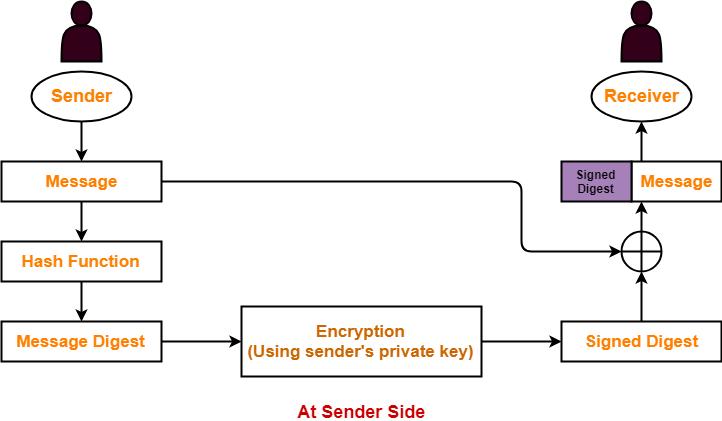## At Receiver Side-

• Receiver receives the original message and the signed digest.
• Using a hash function, receiver converts the original message into a message digest.
• Also, receiver decrypts the received signed digest using the sender’s public key.
• On decryption, receiver obtains the message digest.
• Now, receiver compares both the message digests.
• If they are same, then it is proved that the document is coming from the correct entity.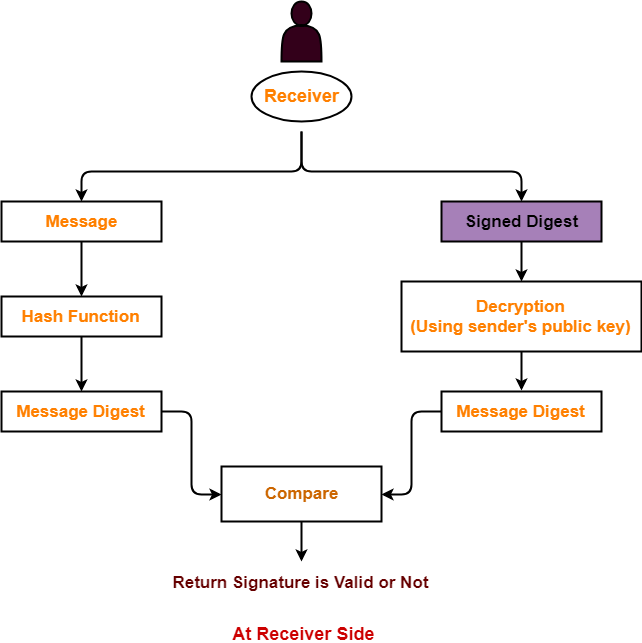Also Read- RSA Algorithm

## Important Points-

### Point-01:

After digitally signing the document, sender sends the following two things to the receiver-

• Signed digest or signature
• Original message

### Point-02:

• Sender uses his private key to digitally sign the document.
• Receiver uses the sender’s public key to verify the signature.

### Point-03:

• Digital signature of a person varies from document to document.
• This ensures authenticity of the document.

### Point-04:

In digital signature,

• There is one to one relationship between a message and a signature.
• Each message has its own signature.

### Point-05:

Digital signature verifies-

• Authenticity
• Integrity
• Non-repudiation

Also Read- Diffie Hellman Key Exchange Algorithm

## Problem-01:

Anarkali digitally signs a message and sends it to Salim. Verification of the signature by Salim requires-

1. Anarkali’s public key
2. Salim’s public key
3. Salim’s private key
4. Anarkali’s private key

## Solution-

Clearly, Option (A) is correct.

## Problem-02:

Consider that B wants to send a message m that is digitally signed to A. Let the pair of private and public keys for A and B be denoted by Kx and Kx+ for x = A, B respectively. Let Kx(m) represent the operation of encrypting m with a key Kx and H(m) represent the message digest. Which one of the following indicates the correct way of sending the message m along with the digital signature to A?

1. {m, KB+(H(m))}
2. {m, KB(H(m))}
3. {m, KA(H(m))}
4. {m, KA+(H(m))}

## Solution-

Clearly, Option (B) is correct.

To gain better understanding about Digital Signatures,

Watch this Video Lecture

Get more notes and other study material of Computer Networks.

Watch video lectures by visiting our YouTube channel LearnVidFun.

## Asymmetric Encryption-

Before you go through this article, make sure that you have gone through the previous article on Asymmetric Key Cryptography.

In asymmetric encryption,

• Sender and receiver use different keys to encrypt and decrypt the message.
• The famous asymmetric encryption algorithms are-In this article, we will discuss about Diffie Hellman Key Exchange Algorithm.

## Symmetric Key Cryptography-

In symmetric key cryptography,

• Both sender and receiver use a common secret key to encrypt and decrypt the message.
• The major issue is exchanging the secret key between the sender and the receiver.
• Attackers might intrude and know the secret key while exchanging it.

Read More- Symmetric Key Cryptography

## Diffie Hellman Key Exchange-

As the name suggests,

• This algorithm is used to exchange the secret key between the sender and the receiver.
• This algorithm facilitates the exchange of secret key without actually transmitting it.

## Diffie Hellman Key Exchange Algorithm-

Let-

• Private key of the sender = Xs
• Public key of the sender = Ys
• Private key of the receiver = Xr
• Public key of the receiver = Yr

Using Diffie Hellman Algorithm, the key is exchanged in the following steps-

### Step-01:

• One of the parties choose two numbers ‘a’ and ‘n’ and exchange with the other party.
• ‘a’ is the primitive root of prime number ‘n’.
• After this exchange, both the parties know the value of ‘a’ and ‘n’.

### Step-02:

• Both the parties already know their own private key.
• Both the parties calculate the value of their public key and exchange with each other.

 Sender calculate its public key as- Ys = aXs mod n Receiver calculate its public key as- Yr = aXr mod n

### Step-03:

• Both the parties receive public key of each other.
• Now, both the parties calculate the value of secret key.

 Sender calculates secret key as- Secret key = (Yr)Xs mod n Receiver calculates secret key as- Secret key = (Ys)Xr mod n

Finally, both the parties obtain the same value of secret key.

## Problem-01:

Suppose that two parties A and B wish to set up a common secret key (D-H key) between themselves using the Diffie Hellman key exchange technique. They agree on 7 as the modulus and 3 as the primitive root. Party A chooses 2 and party B chooses 5 as their respective secrets. Their D-H key is-

1. 3
2. 4
3. 5
4. 6

## Solution-

Given-

• n = 7
• a = 3
• Private key of A = 2
• Private key of B = 5

### Step-01:

Both the parties calculate the value of their public key and exchange with each other.

Public key of A

= 3private key of A mod 7

= 32 mod 7

= 2

Public key of B

= 3private key of B mod 7

= 35 mod 7

= 5

### Step-02:

Both the parties calculate the value of secret key at their respective side.

Secret key obtained by A

= 5private key of A mod 7

= 52 mod 7

= 4

Secret key obtained by B

= 2private key of B mod 7

= 25 mod 7

= 4

Finally, both the parties obtain the same value of secret key.

The value of common secret key = 4.

Thus, Option (B) is correct.

## Problem-02:

In a Diffie-Hellman Key Exchange, Alice and Bob have chosen prime value q = 17 and primitive root = 5. If Alice’s secret key is 4 and Bob’s secret key is 6, what is the secret key they exchanged?

1. 16
2. 17
3. 18
4. 19

## Solution-

Given-

• n = 17
• a = 5
• Private key of Alice = 4
• Private key of Bob = 6

### Step-01:

Both Alice and Bob calculate the value of their public key and exchange with each other.

Public key of Alice

= 5private key of Alice mod 17

= 54 mod 17

= 13

Public key of Bob

= 5private key of Bob mod 17

= 56 mod 17

= 2

### Step-02:

Both the parties calculate the value of secret key at their respective side.

Secret key obtained by Alice

= 2private key of Alice mod 7

= 24 mod 17

= 16

Secret key obtained by Bob

= 13private key of Bob mod 7

= 136 mod 17

= 16

Finally, both the parties obtain the same value of secret key.

The value of common secret key = 16.

Thus, Option (A) is correct.

To gain better understanding about Diffie Hellman Key Exchange Algorithm,

Watch this Video Lecture

Next Article- Digital Signatures

Get more notes and other study material of Computer Networks.

Watch video lectures by visiting our YouTube channel LearnVidFun.

## Cryptography in Network Security-

Before you go through this article, make sure that you have gone through the previous article on Cryptography.

We have discussed-

• Cryptography is a method of storing and transmitting data in a particular form.
• Cryptography techniques are-In this article, we will discuss about Asymmetric Key Cryptography.

## Asymmetric Key Cryptography-

In this technique,

• Sender and receiver use different keys to encrypt and decrypt the message.
• It is called so because sender and receiver use different keys.
• It is also called as public key cryptography.

## Working-

The message exchange using public key cryptography involves the following steps-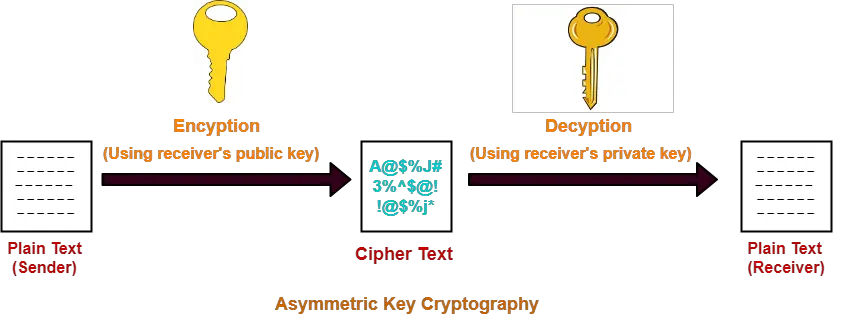## Step-01:

At sender side,

• Sender encrypts the message using receiver’s public key.
• The public key of receiver is publicly available and known to everyone.
• Encryption converts the message into a cipher text.
• This cipher text can be decrypted only using the receiver’s private key.

## Step-02:

• The cipher text is sent to the receiver over the communication channel.

## Step-03:

• Receiver decrypts the cipher text using his private key.
• The private key of the receiver is known only to the receiver.
• Using the public key, it is not possible for anyone to determine the receiver’s private key.
• After decryption, cipher text converts back into a readable format.

The advantages of public key cryptography are-

• It is more robust.
• It is less susceptible to third-party security breach attempts.

The disadvantages of public key cryptography are-

• It involves high computational requirements.
• It is slower than symmetric key cryptography.

## Number of Keys Required-

To use public key cryptography,

• Each individual requires two keys- one public key and one private key.
• For n individuals to communicate, number of keys required = 2 x n = 2n keys.

## Asymmetric Encryption Algorithms-

The famous asymmetric encryption algorithms are-1. RSA Algorithm
2. Diffie-Hellman Key Exchange

## RSA Algorithm-

Let-

• Public key of the receiver = (e , n)
• Private key of the receiver = (d , n)

Then, RSA Algorithm works in the following steps-

## Step-01:

At sender side,

• Sender represents the message to be sent as an integer between 0 and n-1.
• Sender encrypts the message using the public key of receiver.
• It raises the plain text message ‘P’ to the eth power modulo n.
• This converts the message into cipher text ‘C’.

 C = Pe mod n

## Step-02:

• The cipher text ‘C’ is sent to the receiver over the communication channel.

## Step-03:

• Receiver decrypts the cipher text using his private key.
• It raises the cipher text ‘C’ to the dth power modulo n.
• This converts the cipher text back into the plain text ‘P’.

 P = Cd mod n

## NOTE-

### ‘e’ and ‘d’ must be multiplicative inverses modulo Ø(n)

After decryption, receiver must have-

P = Cd mod n

P = (Pe mod n)d mod n

P = Ped mod n

For this equation to be true, by Euler’s Theorem, we must have-

ed = 1 mod Ø(n)

OR

ed = kØ(n) + 1

Thus, e and d must be multiplicative inverses modulo Ø(n).

## Steps to Generate Public Key And Private Key-

An individual can generate his public key and private key using the following steps-

### Step-01:

Choose any two prime numbers p and q such that-

• They are different.
• They are very large.

### Step-02:

Calculate ‘n’ and toilent function Ø(n) where-

• n = p x q
• Ø(n) = (p-1) x (q-1)

### Step-03:

Choose any value of ‘e’ such that-

• 1 < e < Ø(n)
• gcd (e, Ø(n)) = 1

### Step-04:

Determine ‘d’ such that-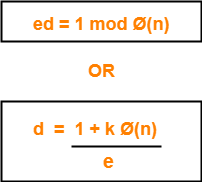• You already know the value of ‘e’ and Ø(n).
• Choose the least positive integer value of ‘k’ which gives the integer value of ‘d’ as a result.
• Use trial and error method.
• Start substituting different values of ‘k’ from 0.

## Problem-01:

In a RSA cryptosystem, a participant A uses two prime numbers p = 13 and q = 17 to generate her public and private keys. If the public key of A is 35, then the private key of A is _______.

## Solution-

Given-

• Prime numbers p = 13 and q = 17
• Public key = 35

### Step-01:

Calculate ‘n’ and toilent function Ø(n).

Value of n,

n = p x q

n = 13 x 17

∴ n = 221

Toilent function,

Ø(n) = (p-1) x (q-1)

Ø(n) = (13-1) x (17-1)

∴ Ø(n) = 192

### Step-02:

• We are already given the value of e = 35.
• Thus, public key = (e , n) = (35 , 221)

### Step-03:

Determine ‘d’ such that-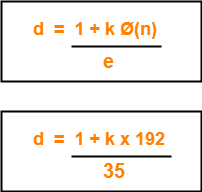Here,

• The least value of ‘k’ which gives the integer value of ‘d’ is k = 2.
• On substituting k = 2, we get d = 11.

Thus, private key of participant A = (d , n) = (11, 221).

## Problem-02:

In the RSA public key cryptosystem, the private and public keys are (e, n) and (d, n) respectively, where n = p x q and p and q are large primes. Besides, n is public and p and q are private. Let M be an integer such that 0 < M < n and f(n) = (p-1)(q-1).

Now consider the following equations-

I. M’ = Me mod n and M = (M’)d mod n

II. ed ≡ 1 mod n

III. ed = 1 mod f(n)

IV. M’ = Me mod f(n) and M = (M’)d mod f(n)

Which of the above equations correctly represent RSA cryptosystem?

1. I and II
2. I and III
3. II and IV
4. III and IV

## Solution-

Clearly, Option (B) is correct.

To gain better understanding about RSA Algorithm,

Watch this Video Lecture

Next Article- Diffie Hellman Key Exchange Algorithm

Get more notes and other study material of Computer Networks.

Watch video lectures by visiting our YouTube channel LearnVidFun.

## Cryptography in Network Security-

In network security,

• Cryptography is a method of storing and transmitting data in a particular form.
• It ensures that only the person for whom the message is intended can read the message.

The message exchange using cryptography involves the following steps-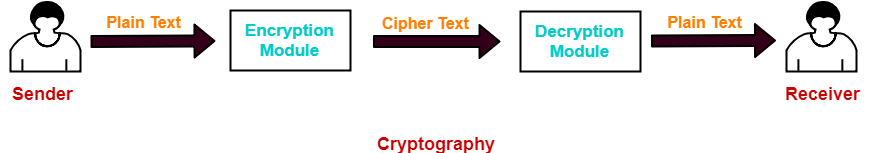## Step-01:

At sender side,

• Using an encryption algorithm, the message is converted into an unreadable form.
• The message in unreadable form is called as cipher text.

## Step-02:

• The cipher text is sent to the receiver over the communication channel.
• Since the message is encrypted, the attackers can not read the message.

## Step-03:

• Using a decryption algorithm, the message is again converted into the readable form.
• Then, receiver can read the message.

## Cryptography Techniques-

Cryptography techniques may be classified as-1. Symmetric Key Cryptography
2. Asymmetric Key Cryptography

In this article, we will discuss about symmetric key cryptography.

## Symmetric Key Cryptography-

In this technique,

• Both sender and receiver uses a common key to encrypt and decrypt the message.
• This secret key is known only to the sender and to the receiver.
• It is also called as secret key cryptography.

## Working-

The message exchange using symmetric key cryptography involves the following steps-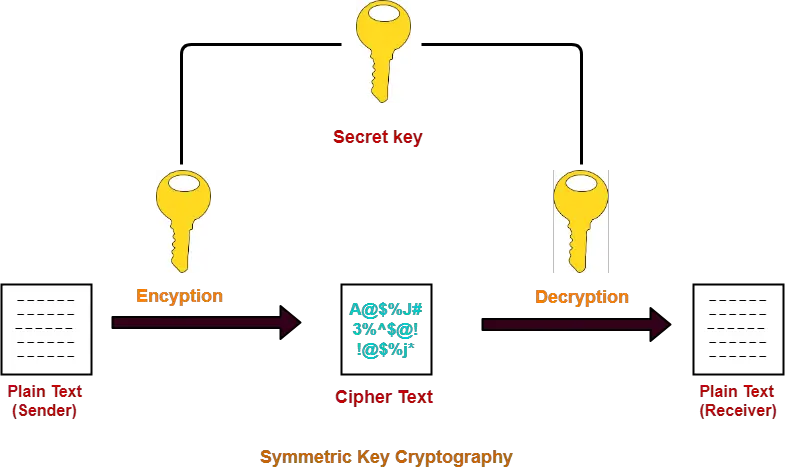• Before starting the communication, sender and receiver shares the secret key.
• This secret key is shared through some external means.
• At sender side, sender encrypts the message using his copy of the key.
• The cipher text is then sent to the receiver over the communication channel.
• At receiver side, receiver decrypts the cipher text using his copy of the key.
• After decryption, the message converts back into readable format.

## Symmetric Encryption Algorithms-

Some of the encryption algorithms that use symmetric key are-

• Advanced Encryption Standard (AES)
• Data Encryption Standard (DES)

The advantages of symmetric key algorithms are-

• They are efficient.
• They take less time to encrypt and decrypt the message.

## Point-01:

 The number of keys required is very large.

In symmetric key cryptography,

• Each pair of users require a unique secret key.
• If N people in the world wants to use this technique, then there needs to be N(N-1) / 2 secret keys.
• For 1 million people to communicate, a half billion secret keys would be needed.

### How N(N-1)/2 Keys Will Be Required?

• Consider a complete graph with N nodes.
• Consider each node represents one person.
• Then, each person will require (N-1) keys to communicate with other (N-1) people.
• Thus, each edge must have a unique key for communication.
• Thus, Number of keys required = Number of edges = nC2 = n(n-1)/2.

## Point-02:

• Sharing the secret key between the sender and receiver is an important issue.
• While sharing the key, attackers might intrude.

 To overcome this disadvantage, Diffie Hellman Key Exchange Algorithm is used for exchanging the secret key.

## Point-01:

In symmetric key cryptography,

• Both sender and receiver uses the same key.
• Sender encrypts the message using his copy of the key.
• Receiver decrypts the message using his copy of the key.
• The key must not be known to anyone else other than sender and receiver.
• If the secret key is known to any intruder, he could decrypt the message.

## Point-02:

• This cryptography technique is called as symmetric key cryptography.
• It is because both sender and receiver use the same key on their sides.

## Point-03:

• This cryptography technique is called as secret key cryptography.
• It is because the key has to be kept secret between the sender and receiver.

To gain better understanding about Symmetric Key Cryptography,

Watch this Video Lecture

Next Article- Asymmetric Key Cryptography | RSA Algorithm

Get more notes and other study material of Computer Networks.

Watch video lectures by visiting our YouTube channel LearnVidFun.